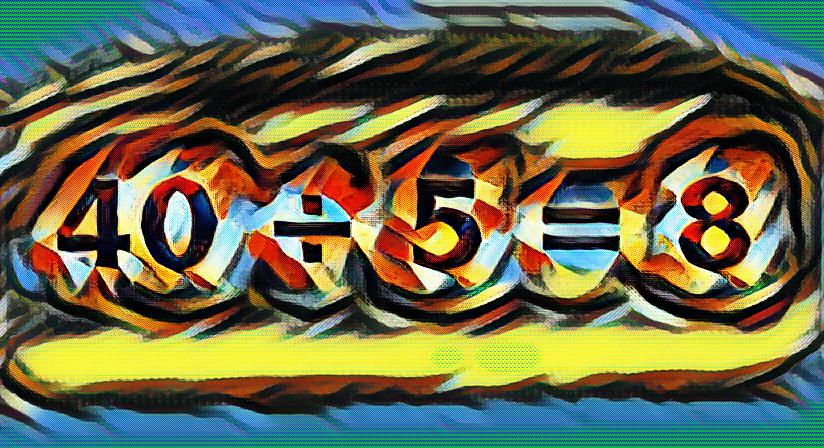# What is a quotient?

In science there are two answers to the question. The first is that a quotient is a result obtained by dividing one quantity by another. The second, more generic definition is a degree or amount of a specified quality or characteristic – or the ratio of two reference values.

## Quotient in mathematics

In math theory, the quotient is part of division. There are three originating elements of division:

• Divisor
• Dividend
• Quotient

For example, when dividing a dividend such as the number ten by the divisor number two, the quotient or answer is five.

In this example, the divisor and dividend are even numbers. However, quotients do not always result in even numbers. As an example, if the divisor is 4 and the dividend is 20, the quotient will be 5.

In algebraic math, it is written differently. In this case a slash (/) or division symbol (÷) divides the dividend from the divisor. An equal sign (=) follows the quotient.

Example: 40 ÷ 5 = 8
Example: 40 / 5 = 8

Where the number 40 is the dividend and 5 is the divisor, 8 is the resulting quotient.

## Quotient used in science

A quotient can be used as the degree or amount of a specified quality or property. The word “quotient” comes from Latin, “quotient”, which means “how often”.

To use the word “quotient” in a sentence to indicate degree or amount, one might ask: “What is the quotient of last year’s population census according to demographics?”

Scientific equations in chemistry are often used to determine chemical reactions. Here, too, a quotient represents the ratio of two reference values.

Quotients are also found in engineering and physics terminology due to the fact these disciplines also use formulas and equations.